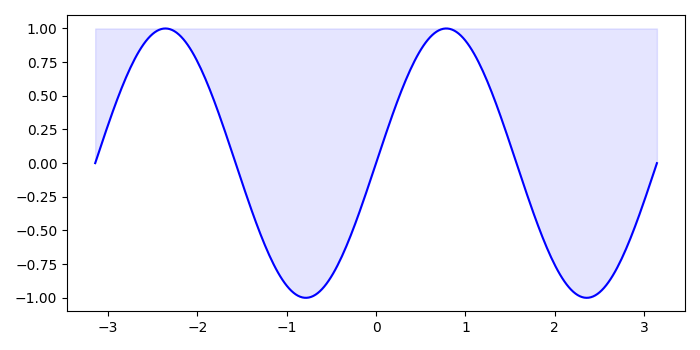# How to fill color above the curve in Matplotlib Program?

To fill color above the curve, we can take the following steps −

## Steps

• Initialize the variable n. Initialize and data points using numpy.

• Create a figure and a set of subplots, fig and ax.

• Plot the curve using plot() method.

• Using fill_between() method, fill the area between two curves, with 1 value.

• To display the figure, use show() method.

## Example

import numpy as np
import matplotlib.pyplot as plt
plt.rcParams["figure.figsize"] = [7.00, 3.50]
plt.rcParams["figure.autolayout"] = True
n = 256
X = np.linspace(-np.pi, np.pi, n, endpoint=True)
Y = np.sin(2 * X)
fig, ax = plt.subplots()
ax.plot(X, Y, color='blue', alpha=1.00)
ax.fill_between(X, Y, 1, color='blue', alpha=.1)
plt.show()

## Output